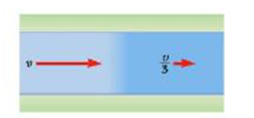Chapter 9, Problem 7CQ

Chapter
Section
Textbook Problem

Water flows along a streamline down a river of constant width (Fig. CQ9.7). Over a short distance the water slows from speed v to v/3. Which of the following can you correctly conclude about the river’s depth? (a) It became deeper by a factor of 32. (b) It became shallower by a factor of 3. (c) It became deeper by a factor of 32. (d) It became shallower by a factor of 32.Figure CQ9.7

To determine
The nature of river’s depth.

Explanation

From equation of continuity,

A1v1=A2v2

• A1 and A2 are the cross-sectional areas.
• v1 and v2 are the speeds

Still sussing out bartleby?

Check out a sample textbook solution.

See a sample solution

The Solution to Your Study Problems

Bartleby provides explanations to thousands of textbook problems written by our experts, many with advanced degrees!

Get Started

Little fat digestion takes place in the stomach. T F

Nutrition: Concepts and Controversies - Standalone book (MindTap Course List)

What are genomes?

Human Heredity: Principles and Issues (MindTap Course List)

For A = 3i + j + k, B = -i + 2j + 5k, and C = 2j - 3k, find C (A - B).

Physics for Scientists and Engineers, Technology Update (No access codes included)